﻿ 数字图像相关法测量局域变形场中形函数和模板尺寸的影响
«上一篇文章快速检索 高级检索

 力学学报2015, Vol. 47Issue (5): 848-862  DOI: 10.6052/0459-1879-15-1190

### 引用本文 [复制中英文]

[复制中文]
Xu Xiaohai, Su Yong, Cai Yulong, Cheng Teng, Zhang Qingchuan. INFLUENCE OF SHAPE FUNCTIONS AND TEMPLATE SIZE IN DIGITAL IMAGE CORRELATION METHOD FOR HIGHLY INHOMOGENEOUS DEFORMATIONS[J]. Chinese Journal of Ship Research, 2015, 47(5): 848-862. DOI: 10.6052/0459-1879-15-119.
[复制英文]

### 文章历史

2015-04-09 收稿
2015-06-15 录用
2015–06–18 网络版发表

1 形函数

 $\left[{\begin{array}{*{20}{c}} {x'}\\ {y'} \end{array}} \right] = \left[{\begin{array}{*{20}{c}} 1&0&u\\ 0&1&v \end{array}} \right]\;\left[{\begin{array}{*{20}{c}} x\\ y\\ 1 \end{array}} \right]$ (1)

 $\left[{\begin{array}{*{20}{c}} {x'}\\ {y'} \end{array}} \right] = \left[{\begin{array}{*{20}{c}} {1 + {u_x}}&{{u_y}}&u\\ {{v_x}}&{1 + {v_y}}&v \end{array}} \right]\;\left[{\begin{array}{*{20}{c}} x\\ y\\ 1 \end{array}} \right]$ (2)

 $\left[{\begin{array}{*{20}{c}} {x'}\\ {y'} \end{array}} \right] = \;\left[{\begin{array}{*{20}{c}} {\frac{1}{2}{u_{xx}}}&{{u_{xy}}}&{\frac{1}{2}{u_{yy}}}&{1 + {u_x}}&{{u_y}}&u\\ {\frac{1}{2}{v_{xx}}}&{{v_{xy}}}&{\frac{1}{2}{v_{yy}}}&{{v_x}}&{1 + {v_y}}&v \end{array}} \right]\;\left[{\begin{array}{*{20}{c}} {{x^2}}\\ {xy}\\ {{y^2}}\\ x\\ y\\ 1 \end{array}} \right]$ (3)

2 拉伸实验 2.1 实验装置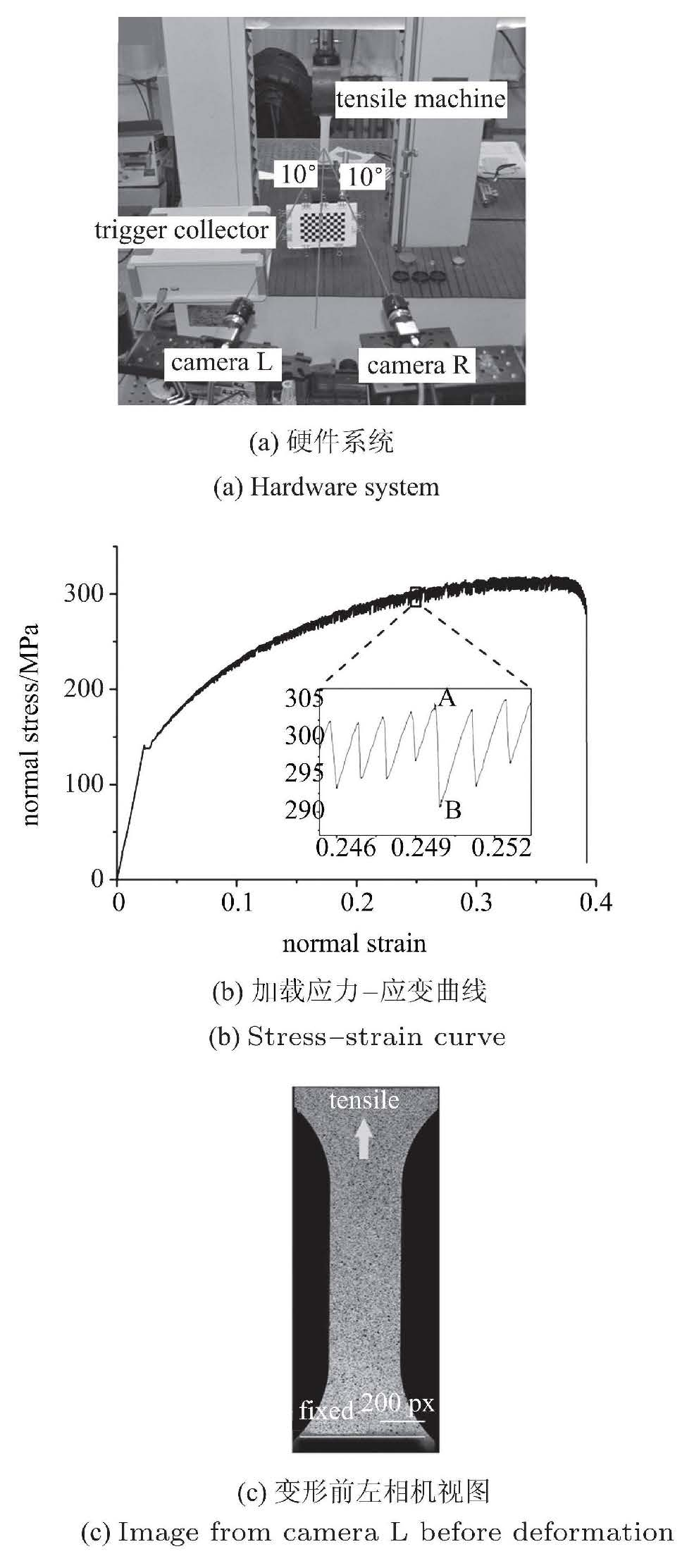图 1 拉伸实验装置和数据 Fig.1 Experimental setup and data
2.2 计算结果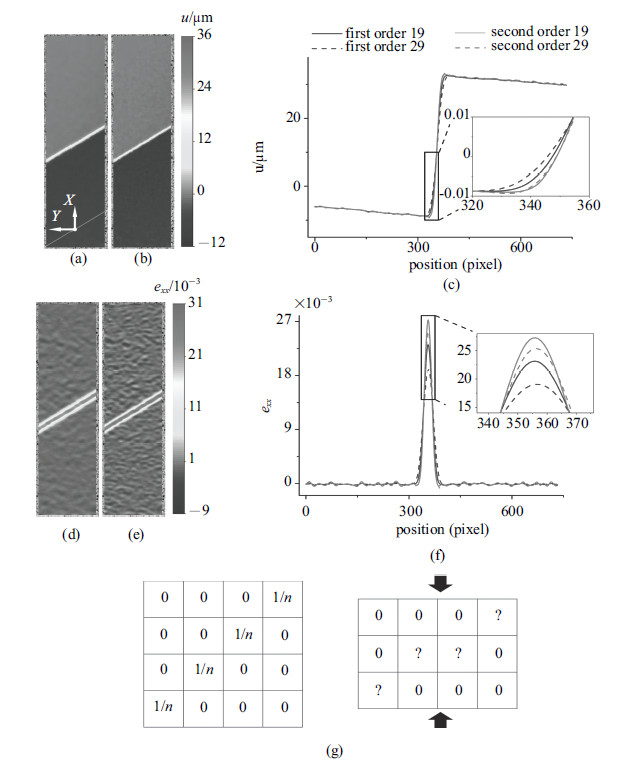图 2 不同形函数和模板尺寸计算得到的位移和应变. 一阶和二阶形函数得到的拉伸方向(a), (b)位移场和(d), (e)应变场. (c)位移曲线. (f)应变曲线. (g)传统平滑模板 (左)和等效平滑模板 (右) Fig.2 Displacement and strain fields and curves with different shape functions and templates. (a), (b) Full field displacement $u$ and (d), (e) strain $e_{xx}$ using first- (left) and second-order (right) shape functions. (c) Smoothed displacement curves and (f) smoothed strain curves. (g) Traditional average template (left) and equivalent template (right)
3 数值模拟 3.1 非线性拟合

 ${e_{xx}}(x) = a{e^{ - \frac{{{{(x - b)}^2}}}{{2{c^2}}}}} + d$ (4)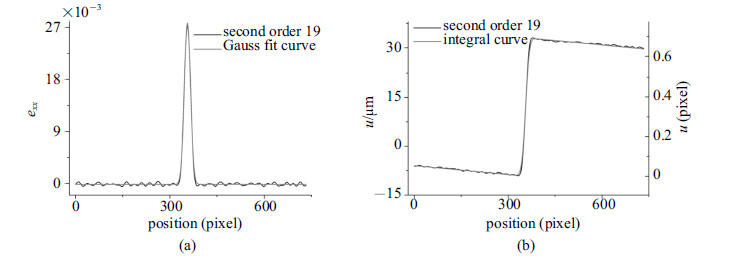图 3 测量与拟合结果. (a)应变曲线及其高斯拟合曲线. (b)位移曲线及 (a)中拟合曲线的积分曲线 Fig.3 Experimental setup and data. (a) Smoothed strain curve and its Gaussian fit curve and (b) smoothed displacement curve and the integral curve whose derivative is the fit (a)
 ${W_{{\rm{HM}}}} = 2\sqrt {2\ln 2} c$ (5)

 $u(x) = ac\sqrt {\frac{\pi }{2}} [{\rm{erf}}\left( {\frac{{x\; - \;b}}{{\sqrt 2 c}}} \right) + {\rm{erf}}\left( {\frac{b}{{\sqrt 2 c}}} \right)] + dx$ (6)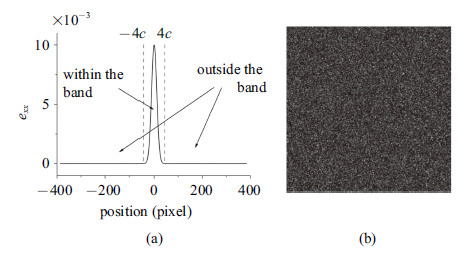图 4 (a)波特文-勒夏特利埃带带内和带外的划分, (b)模拟生成的参考图像 Fig.4 Area within and outside the band (a) and simulated reference speckle image (b)
3.2 模拟散斑图像

 $u\left( x \right) = ac\sqrt {\frac{\pi }{2}} erf\left( {\frac{x}{{\sqrt 2 c}}} \right)$ (7)
 $e(x) = a{{\rm{e}}^{ - \frac{{{x^2}}}{{2{c^2}}}}}$ (8)

 ${E_{{\rm{FFDE}}}} = \sqrt {\frac{{\sum\limits_{i = 1}^M {\sum\limits_{j = 1}^N {{{\left( {{u_{ij}}\; - \;u_{ij}^t} \right)}^2}} } }}{{MN}}}$ (9)

 ${E_{{\rm{SDE}}}} = \sqrt {\frac{{\sum\limits_{i = 1}^M {{{\left( {\sum\limits_{j = 1}^N {{u_{ij}}} /N\; - \;u_i^t} \right)}^2}} }}{M}}$ (10)

4 讨 论 4.1 变形固定时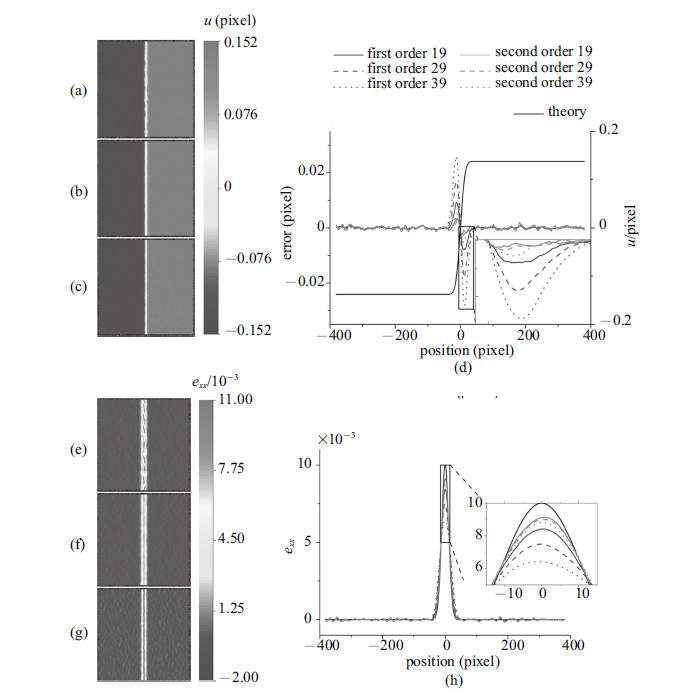图 5 不同形函数和模板尺寸得到的拉伸方向的(a)$\sim$(d)位移和(e)$\sim$(h)应变 Fig.5 Displacement (a)$\sim$(d) and strain (e)$\sim$(h) fields and (error) curves using different shape functions and templates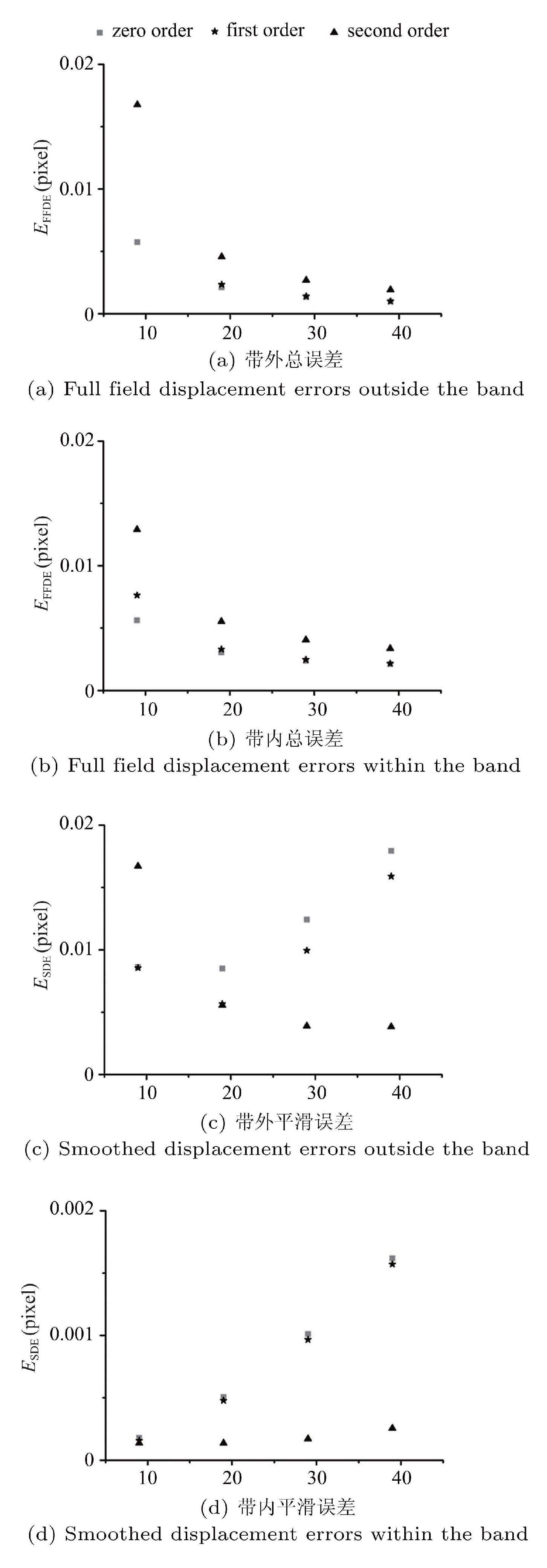图 6 带外和带内的位移总误差和位移平滑误差 Fig.6 Displacement errors within and outside the band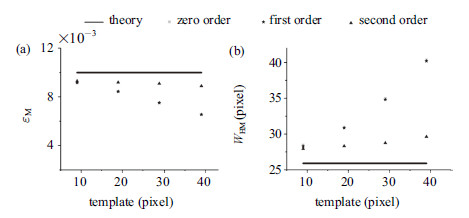图 7 带内最大应变(a)和带宽(b)受形函数和模板尺寸的影响 Fig.7 Maximum strain value within the band (a) and full width (b) at half maximum of different shape functions and templates

4.2 仅带内最大应变$a$改变

4.1节针对固定的变形讨论了形函数和模板对计算结果的影响,本小节取出3.2节中生成的第一组散斑图像 (带内最大应变$a$改变)来讨论不同应变梯度下,形函数和模板对计算结果的影响.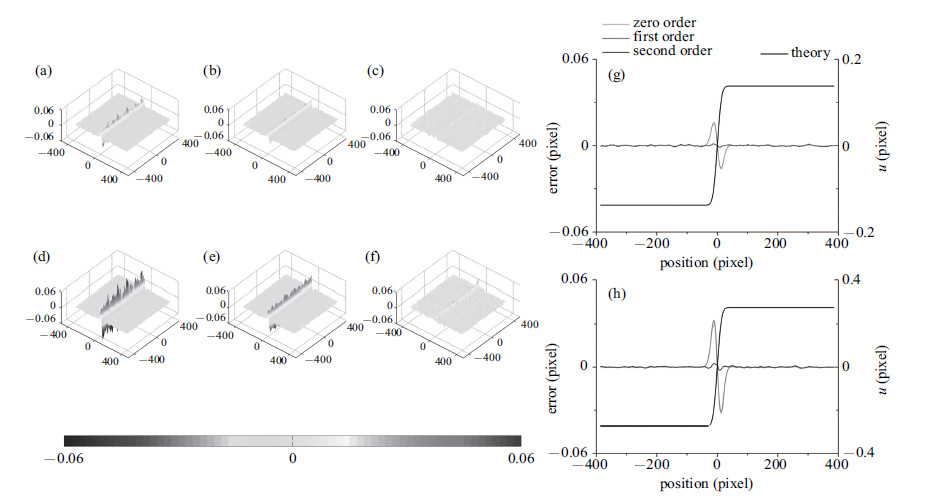图 8 不同带内最大应变时的位移误差. (a)$\sim$(c) $a = 0.01$. (e)$\sim$(g) $a =0.02$时3种形函数的位移误差分布.(d) $a=0.01$. (h) $a = 0.02$时的理论位移曲线和位移误差曲线 Fig.8 Displacement errors of different maximum strain, within the band. (a)$\sim$(c) $a = 0.01$ and (e)$\sim$(g) $a = 0.02$, full field displacement errors calculated using 3 shape functions. (d) $a = 0.01$ and (h) $a = 0.02$, theoretical displacement curve and smoothed\\ displacement error curves using 3 shape functions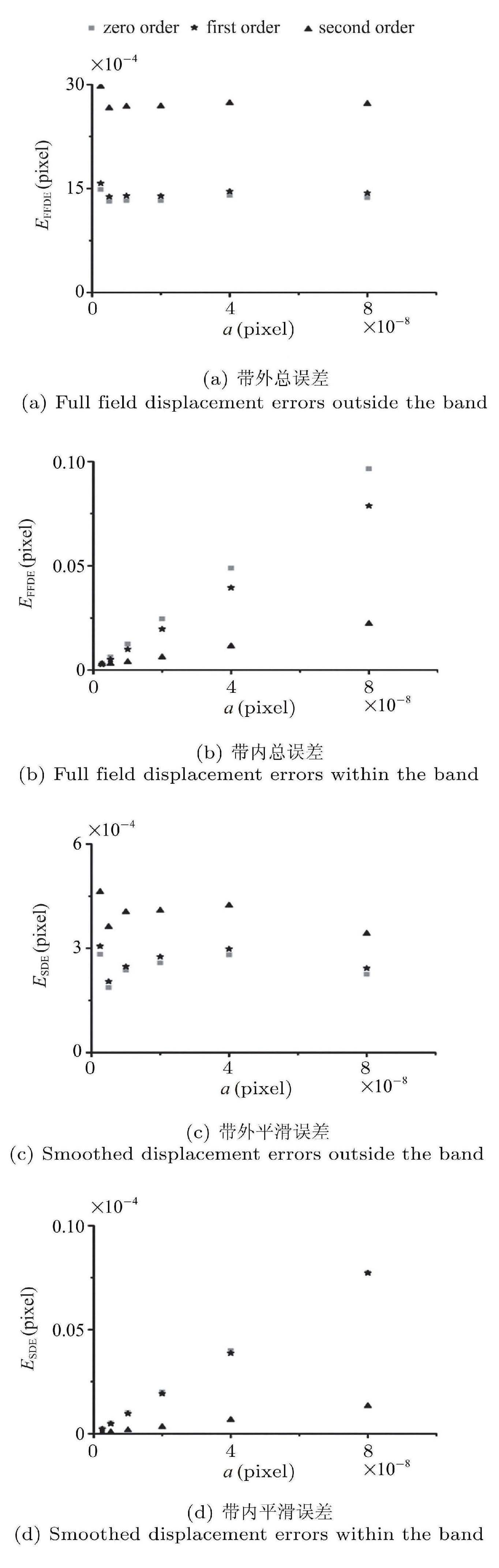图 9 不同带内最大应变时, 带内和带外的位移总误差和平滑误差 Fig.9 Displacement statistical errors of different maximum strain within the band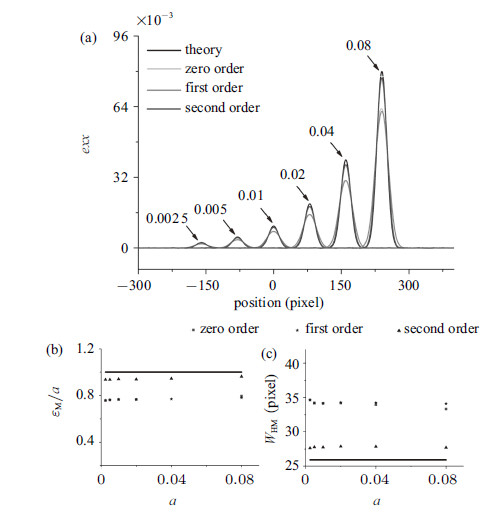图 10 不同带内最大应变时的应变曲线及其拟合参数 Fig.10 Strain curves and the fitting parameters of different maximum strains within the band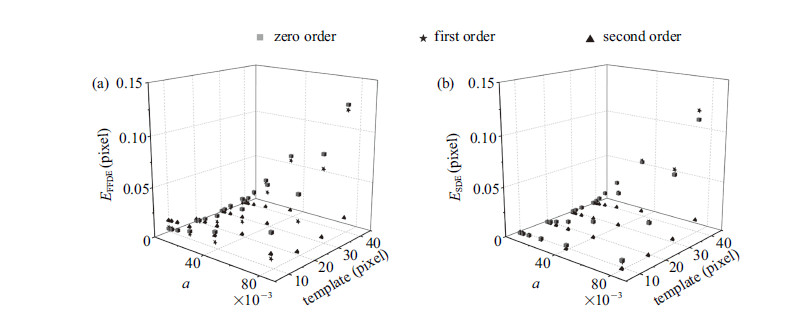图 11 不同带内最大应变和模板尺寸时, 3种形函数得到的带内位移误差. (a)带内位移总误差. (b)带内位移平滑误差 Fig.11 Displacement errors of different maximum strains within the band and templates. (a) Full field displacement errors within the band. (b) Smoothed displacement errors within the band

4.3 仅带宽相关量$c$改变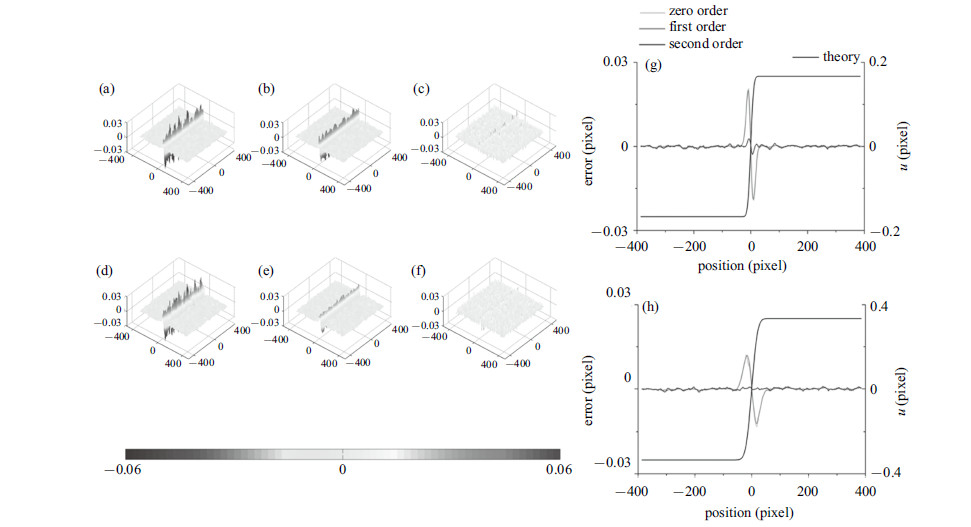图 12 不同带宽时的位移误差. (a)$\sim$(c) $c= 8$像素. (e)$\sim$(g) $c = 16$像素时3种形 函数的位移误差分布. (d) $c = 8$.(h) $c = 16$像素时的理论位移曲线和位移误差曲线 Fig.12 Displacement errors of different band widths. (a)$\sim$(c) $c = 8$ pixel and (e)$\sim$(g) $c = 16$ pixel, full field displacement errors calculated using 3 shape functions. (d) $c = 8$ pixel and (h) $c = 16$ pixel, theoretical displacement curve and smoothed displacement error curves using 3 shape functions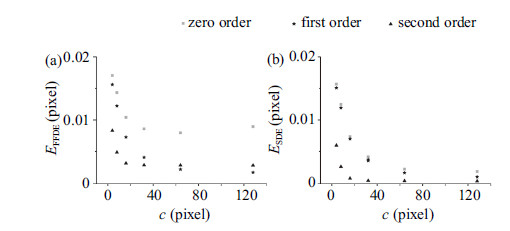图 13 不同带宽时带内位移误差 Fig.13 Displacement statistical errors of different band widths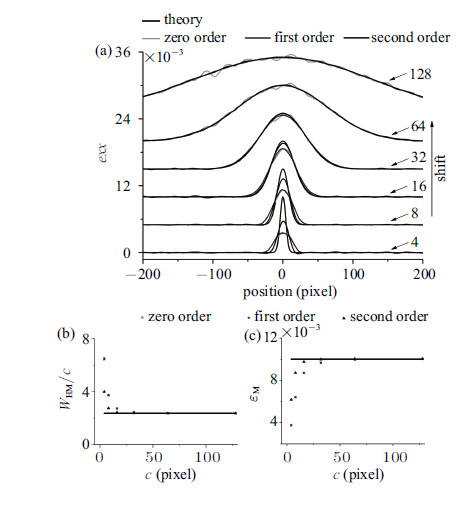图 14 不同带宽时的应变曲线及其拟合参数 Fig.14 Strain curves and the fitting parameters of different band widths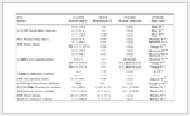表 1 带内最大应变的误差 Table 1 Errors of the maximum strain within the band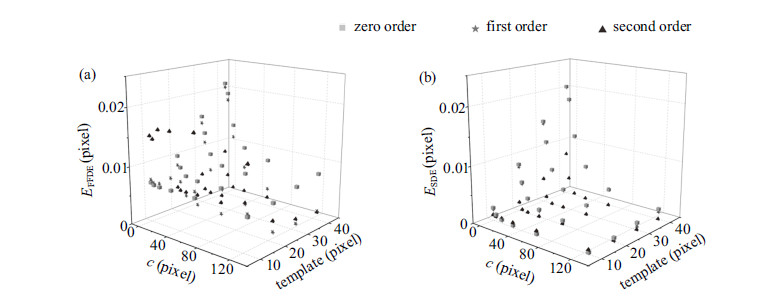图 15 不同带宽相关量和模板尺寸时的带内位移误差. (a)带内位移总误差. (b)带内位移平滑误差 Fig.15 Displacement errors of different band widths and templates. (a) Full field displacement errors within the band.(b) Smoothed displacement errors within the band

5 其他实验影响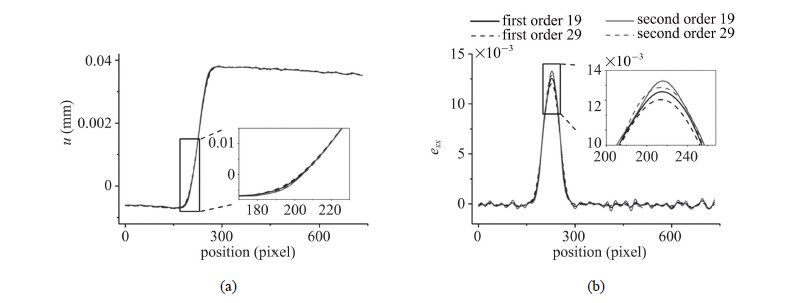图 16 验证实验得到的 (a)位移曲线和 (b)应变曲线 Fig.16 Smoothed displacement curves (a) and strain curves (b)
6 结 论

(1)在均匀变形区,一阶和二阶形函数的计算结果相当 (但对于二阶的形函数需要更大的模板才能获得与一阶形函数相当的随机 误差);

(2)在局域变形场,模板尺寸相同时二阶形函数较一阶形函数有明显的优势 (二阶形函数欠匹配带来的系统误差较小,而随机误差相较 于系统误差是高阶小量).

(1)数值模拟揭示了在局域变形测量中,形函数和模板尺寸等计算参量对数字图像相关法计算结果的影响:

(a)二阶形函数有较大的临界模板并且在模板较大时,能获得比一阶形函数更好的结果;

(b)带内最大应变与位移误差和应变误差均成正比,且对波特文-勒夏特利埃带的带宽无影响;

(c)二阶形函数在波特文-勒夏特利埃带带宽较小时能得到更好的结果,但在带宽很大时的结果反而更差.

(2)为了判断数字图像相关法测量结果的可靠性,提出了当一阶和二阶形函数相对误差小于10%时,二阶形函数阶数结果是 可靠的判据. 该判据是通过特定的局域变形场(波特文-勒夏特利埃带)分析得到的,且受模板尺寸制约. 其他局域变形场(比如试件缺口附近和裂纹尖端),也属于高阶变形,但变形形式与波特文-勒夏特利埃带不同,分析的结果随之改变,在考虑二阶以上变形影响较小时,上述判据同样适用. 我们下一步研究工作将针对像素应变梯度'' (即每像素的应变梯度)进行讨论,以期得到定量的、普适的判据.

(3)实验和数值模拟的计算结果表明,可以通过设置数字图像相关法的算法参量来获得更为准确的结果;

(4)在局域变形测量中,建议采用二阶形函数和较大 (降低随机误差)的模板尺寸; 如果要采用一阶形函数,需要采用较小(降低系统误差)的模板尺寸;

(5)对于测量结果不满足该判据的情况 (即一阶和二阶形函数计算结果的带内最大应变相对误差大于10%),给出了通过提高采样频率和空间分辨率来获取可靠结果的方法.

  Peters WH, Ranson WF. Digital imaging techniques in experimental stress analysis. Optics Engineering, 1981, 21: 427-431  Schreier H, Orteu JJ, Sutton MA. Image correlation for shape, motion and deformation measurements: Basic concepts, theory and applications. New York: Springer, 2009  李喜德, 谭玉山. 数字散斑强度相关计量的特性研究. 光学学报, 1992, 12 (12): 1135-1139 (Li Xide, Tan Yushan. Investigation of the specifics of digital speckle intensity correlation metering. Acta Optica Sinica, 1992, 12(12): 1135-1139 (in Chinese))  潘兵, 谢惠民, 续伯钦等. 数字图像相关中的亚像素位移定位算法发展. 力学进展, 2005, 35 (3): 345-352 (Pan Bing, Xie Huimin, Xu Boqin, et al. Development of sub-pixel displacements registration algirithms in digital image correlation. Advances in Mechanics, 2005, 35(3): 345-352 (in Chinese))  Zhang ZF, Kang YL, Wang HW, et al. A novel coarse-fine search scheme for digital image correlation method. Measurement, 2006, 39(8): 710-718  杨勇, 王琰蕾, 李明等. 高精度数字图像相关测量系统及其技术研究. 光学学报, 2006, 26 (2): 197-201 (Yang Yong, Wang Yanlei, Li Ming, et al. Research of high-accuray digital image correlation measurement system. Acta Optica Sinica, 2006, 26(2): 197-201 (in Chinese))  潘兵, 谢惠明, 戴福隆. 数字图像相关中亚像素位移测量算法的研究. 力学学报, 2007, 39 (2): 245-252 (Pan Bing, Xie Huimin, Dai Fulong. An investigation of sub-pixel displacements registeration algorithms in digital image correlation. Journal of Theoretical and Applied Mechanics, 2007, 39(2): 245-252 (in Chinese))  Li XD, Deng B, Shi HJ. Displacement measurements in micro-region with generalized digital speckle correlation method. Proc. SPIE, 2002, 4537: 366-369  王怀文, 亢一澜, 谢和平. 数字散斑相关方法与应用研究进展. 力学进展, 2005, 35 (2): 195-203 (Wang Huaiwen, Kang Yilan, Xie Heping. Advance in digital speckle correlation and its application. Advances in Mechanics, 2005, 35(2): 195-203 (in Chinese))  潘兵, 谢惠民, 续伯钦等. 应用数字图像相关方法测量含缺陷试样的全场变形. 实验力学, 2007, 22 (3-4): 379-384 (Pan Bing, Xie Huimin, Xu Boqin, et al. Full-field deformation measurement of specimen with discontinuity using digital image correlation. Journal of Experimental Mechanics, 2007, 22(3-4): 379-384 (in Chinese))  曾祥福, 刘程林, 马少鹏. 高速三维数字图像相关系统及其动载三维变形测量. 北京理工大学学报, 2012, 32 (4): 364-369 (Zeng Xiangfu, Liu Chenglin, Ma Shaopeng. Measurement of dynamic three-dimensional deformation of structures using high-speed 3-D digital image correlation system. Transactions of Beijing Institute if Technology, 2012, 32(4): 364-369 (in Chinese))  Kang YL, Zhang ZF, Wang HW, et al. Experimental investigations of the effect of thickness on fracture toughness of metallic foils. Materials Science and Engineering A, 2005, 394(1-2): 312-319  陈琳, 卢荣胜. 数字图像相关法测量含缺陷试样全场变形量. 激光杂志, 2014, 35 (11): 16-19 (Chen Lin, Lu Rongsheng. Digital image correlation method for full-field deformation measurement of specimen with discontinuity. Laser Journal, 2014, 35(11): 16-19 (in Chinese))  朱珍德, 张勇, 李术才等. 用数字图像相关技术进行红砂岩细观裂纹损伤特效研究. 岩石力学与工程学报, 2015, 24 (7): 1123-1128 (Zhu Zhende, Zhang Yong, Li Shucai, et al. Studies on microcosmic crack damage properties of a red sandstone with digital image technique. Chinese Journal of Rock Mechanics and Engineering, 2005, 24(7): 1123-1128 (in Chinese))  刘聪, 陈振宁, 何小元. 3D-DIC在土木结构力学性能试验研究中的应用. 东南大学学报 (自然科学版), 2014, 44 (2): 339-344 (Liu Cong, Chen Zhenning, He Xiaoyuan. Application of 3D-DIC in experimental study on mechanical properties of civil structures. Journal of Southeast University (Nature Science Edition), 2014, 44(2): 339-344 (in Chinese))  蔡玉龙, 符师桦, 王玉辉等. 基于三维数字图像相关法的5456铝镁合金锯齿形屈服研究. 金属学报, 2014, 50 (12): 1491-1497 (Cai Yulong, Fu Shihua, Wang Yuhui, et al. Serrated yielding of 5456 aluminium magne sium alloy based on three dimensional digital image correlation. Acta Metallurgica Sinica, 2014, 50(12): 1491-1497 (in Chinese))  Gao Y, Cheng T, Su Y, et al. High-efficiency and high-accuracy digital image correlation for three-dimensional measurement. Optics Lasers in Engineering, 2015, 65: 73-80  Zhang QC, Toyooka S, Meng ZB, et al. Investigation of slipband propagation in aluminum alloy with dynamic speckle interferometry. SPIE, 1999, 3585: 389-398  Zhang QC, Jiang ZY, Jiang HF, et al. On the propagation and pulsation of Portevin-Le Chatelier deformation bands: An experimental study with digital speckle pattern metrology. International Journal of Plasticity, 2000, 40(2): 393-400  Schreier HW, Sutton MA. System errors in digital image correlation due to undermatched subset shape functions. Experimental Mechanics, 2002, 42(3): 303-310  王学滨, 杜亚志, 潘一山. 考虑一阶和二阶位移梯度的数字图像相关方法在剪切带测量中的比较. 工程力学, 2013, 30 (7): 282-297 (Wang Xuebin, Du Yazhi, Pan Yishan. A comparsion of DIC methods considering the first- and second-order displacement gradients in shear band measurements. Engineering Mechanics, 2013, 30(7): 282-297 (in Chinese))  Bornert M, Brémand F, Doumalin P, et al. Assessment of digital image correlation measurement errors: Methodology and results. Exp Mech , 2009, 49(3): 353-370  Yu LP, Pan B. The errors in digital image correlation due to overmatched shape functions. Meas Sci Technol , 2015, 26: 045202  Gates M, Gonzalez J, Lambros J. Subset refinement for digital volume correlation: Numerical and experimental applications. Exp Mech , 2015, 55(1): 245-259  Pan B, Xie HM, Wang ZY, et al. Study on subset size selection in digital image correlation for speckle patterns. Optics Express, 2008, 16(10): 7037-7048  Lu L, Wang Z, Vo M, et al. Accuracy enhancement of digital image correlation with B-spline interpolation. Opt. Lett., 2011, 36: 3070-3072  Lu H, Cary PD. Deformation measuement by digital image correlation: implemention of a second-order displacement gradient. Experimental Mechanics, 2000, 40(4): 393-400  Sutton MA, Yan JH, Tiwari V, et al. The effect of out-of-plane motion on 2D and 3D digital image correlation measurements. Optics Lasers in Engineering, 2008, 46 (10): 746-757  Cheng T, Xu XH, Cai YL, et al. Investigation of Portevin-Le Chatelier effect in 5456 Al-based alloy using digital image correlation. Optics Lasers in Engineering, 2015, 65: 89-92  Zhou P, Goodson KE. Subpixel displacement and deformation gradient measurement using digital image/speckle correlation (DISC). Optics Engineering, 2001, 40(8): 1613-1620
INFLUENCE OF SHAPE FUNCTIONS AND TEMPLATE SIZE IN DIGITAL IMAGE CORRELATION METHOD FOR HIGHLY INHOMOGENEOUS DEFORMATIONS
Xu Xiaohai, Su Yong, Cai Yulong, Cheng Teng, Zhang QingchuanDepartment of Modern Mechanics, University of Science and Technology of China, CAS Key Laboratory of Mechanical Behavior and Design of Materials, Heifei 230027, China
Fund: The project was supported by the National Basic Research Program of China (2011CB302105)and the National Natural Science Foundation of China (11372300, 11332010, 51271174, 11127201, 11472266 and 11428206).
Abstract: Digital image correlation (DIC) method and its reliability and accuracy are commonly accepted in the measurements of affine deformations since it obtains comparable results with strain gauges. However, in engineering measurements, there are always substantial local deformations with high strain gradients such as the Portevin-Le Chatelier (PLC) bands, deformations near gaps and crack tips. In these situations, because the results within the contact areas are smoothed, strain gauges are restricted. Here DIC method can be employed to solve this problem. Problems that the calculation parameters (the order of shape functions, template size, etc.) seriously impact on the results and often make it difficult to get reliable results. In this paper, the deep mechanism that how the shape functions and the size of the templates impact on the accuracy of DIC results is discovered via analyzing PLC bands with different gradients in tensile tests and simulated bands. Second-order shape functions are more suitable than first-order shape functions to describe local deformations according to the simulation results. A gist is proposed that the results of second-order shape functions are reliable and accurate when the relative error between first- and second-order shape functions is less than 10%.
Key words: digital image correlation    local deformations    reliability    shape functions    template size